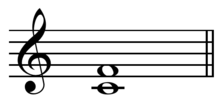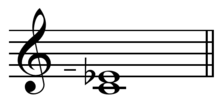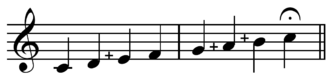# Pythagorean interval

﻿
Pythagorean interval

In musical tuning theory, a Pythagorean interval is a musical interval with frequency ratio equal to a power of two divided by a power of three, or vice versa. For instance, the perfect fifth with ratio 3/2 (equivalent to 31/21) and the perfect fourth with ratio 4/3 (equivalent to 22/31) are Pythagorean intervals.

All the intervals between the notes of a scale are Pythagorean if they are tuned using the Pythagorean tuning system. However, some Pythagorean intervals are also used in other tuning systems. For instance, the above mentioned Pythagorean perfect fifth and fourth are also used in just intonation.

## Interval table

Name Short Other name(s) Ratio Factors Derivation Cents ET
Cents
MIDI file Fifths
diminished second d2 524288/531441 219/312 -23.460 0 -12
(perfect) unison P1 1/1 1/1 0.000 0 0
Pythagorean comma 531441/524288 23.460 0 12
minor second m2 limma,
diatonic semitone,
minor semitone
256/243 28/35 90.225 100 -5
augmented unison A1 apotome,
chromatic semitone,
major semitone
2187/2048 37/211 113.685 100 7
diminished third d3 tone,
whole tone,
whole step
65536/59049 216/310 180.450 200 -10
major second M2 9/8 32/23 3·3/2·2 203.910 200 2
semiditone m3 (Pythagorean minor third) 32/27 25/33 294.135 300 -3
augmented second A2 19683/16384 39/214 317.595 300 9
diminished fourth d4 8192/6561 213/38 384.360 400 -8
ditone M3 (Pythagorean major third) 81/64 34/26 27·3/16·2 407.820 400 4
perfect fourth P4 diatessaron,
sesquitertium
4/3 22/3 2/3 498.045 500 -1
augmented third A3 177147/131072 311/217 521.505 500 11
diminished fifth d5 tritone 1024/729 210/36 588.270 600 -6
augmented fourth A4 729/512 36/29 611.730 600 6
diminished sixth d6 262144/177147 218/311 678.495 700 -11
perfect fifth P5 diapente,
sesquialterum
3/2 3/2 701.955 700 1
minor sixth m6 128/81 27/34 792.180 800 -4
augmented fifth A5 6561/4096 38/212 815.640 800 8
diminished seventh d7 32768/19683 215/39 882.405 900 -9
major sixth M6 27/16 33/24 9·3/8·2 905.865 900 3
minor seventh m7 16/9 24/32 996.090 1000 -2
augmented sixth A6 59049/32768 310/215 1019.550 1000 10
diminished octave d8 4096/2187 212/37 1086.315 1100 -7
major seventh M7 243/128 35/27 81·3/64·2 1109.775 1100 5
augmented seventh A7 (octave − comma) 1048576/531441 1176.540 1200 -12
(perfect) octave P8 diapason 2/1 2/1 1200.000 1200 0
diminished ninth d9 (octave + comma) 531441/262144 312/218 1223.460 1200 12

Notice that the terms ditone and semiditone are specific for Pythagorean tuning, while tone and tritone are used generically for all tuning systems. Interestingly, despite its name, a semiditone (3 semitones, or about 300 cents) can hardly be viewed as half of a ditone (4 semitones, or about 400 cents).Frequency ratio of the 144 intervals in D-based Pythagorean tuning. Interval names are given in their shortened form. Pure intervals are shown in bold font. Wolf intervals are highlighted in red. Numbers larger than 999 are shown as powers of 2 or 3. Other versions of this table are provided here and here.

### 12-tone Pythagorean scale

The table shows from which notes some of the above listed intervals can be played on a instrument using a repeated-octave 12-tone scale (such as a piano) tuned with D-based symmetric Pythagorean tuning. Further details about this table can be found in Size of Pythagorean intervals.Pythagorean perfect fifth on C : C-G (3/2 ÷ 1/1 = 3/2).Pythagorean perfect fifth on D : D-A+ (27/16 ÷ 9/8 = 3/2).Just perfect fourth , one perfect fifth inverted (4/3 ÷ 1/1 = 4/3).Major tone on C : C-D (9/8 ÷ 3/2 = 3/2), two Pythagorean perfect fifths.Pythagorean small minor seventh (1/1 - 16/9) , two perfect fifths inverted.Pythagorean major sixth on C (1/1 - 27/16) , three Pythagorean perfect fifths.Semiditone on C (1/1 - 32/27) , three Pythagorean perfect fifths inverted.Ditone on C (1/1 - 5/4) , four Pythagorean perfect fifths.Pythagorean minor sixth on C (1/1 - 128/81) , four Pythagorean perfect fifths inverted.Pythagorean major seventh on C (1/1 - 243/128) , five Pythagorean perfect fifths.Pythagorean diminished fifth tritone on C (1/1 - 1024/729) , six Pythagorean perfect fifths inverted.

## Fundamental intervals

The fundamental intervals are the superparticular ratios 2/1, 3/2, and 4/3. 2/1 is the octave or diapason (Greek for "across all"). 3/2 is the perfect fifth, diapente ("across five"), or sesquialterum. 4/3 is the perfect fourth, diatessaron ("across four"), or sesquitertium. These three intervals and their octave equivalents, such as the perfect eleventh and twelfth, are the only absolute consonances of the Pythagorean system. All other intervals have varying degrees of dissonance, ranging from smooth to rough.

The difference between the perfect fourth and the perfect fifth is the tone or major second. This has the ratio 9/8, and it is the only other superparticular ratio of Pythagorean tuning, as shown by Størmer's theorem.

Two tones make a ditone, a dissonantly wide major third, ratio 81/64. The ditone differs from the just major third (5/4) by the syntonic comma (81/80). Likewise, the difference between the tone and the perfect fourth is the semiditone, a narrow minor third, 32/27, which differs from 6/5 by the syntonic comma. These differences are "tempered out" or eliminated by using compromises in meantone temperament.

The difference between the minor third and the tone is the minor semitone or limma of 256/243. The difference between the tone and the limma is the major semitone or apotome ("part cut off") of 2187/2048. Although the limma and the apotome are both represented by one step of 12-pitch equal temperament, they are not equal in Pythagorean tuning, and their difference, 531441/524288, is known as the Pythagorean comma.

## Contrast with modern nomenclature

There is a one-to-one correspondence between interval names (number of scale steps + quality) and frequency ratios. This contrasts with equal temperament, in which intervals with the same frequency ratio can have different names (e.g., the diminished fifth and the augmented fourth); and with other forms of just intonation, in which intervals with the same name can have different frequency ratios (e.g., 9/8 for the major second from C to D, but 10/9 for the major second from D to E).Pythagorean diatonic scale on C .

Wikimedia Foundation. 2010.

### Look at other dictionaries:

• Pythagorean comma — (PC) defined in Pythagorean tuning as difference between semitones (A1–m2), or interval between enharmonically equivalent notes (from D♭ to C♯). The di …   Wikipedia

• Pythagorean tuning — is a system of musical tuning in which the frequency relationships of all intervals are based on the ratio 3:2. Its name comes from medieval texts which attribute its discovery to Pythagoras, but its use has been documented as long ago as 3500… …   Wikipedia

• Interval (music) — For the album by See You Next Tuesday, see Intervals (album). Melodic and harmonic intervals.   …   Wikipedia

• Pythagorean theorem — See also: Pythagorean trigonometric identity The Pythagorean theorem: The sum of the areas of the two squares on the legs (a and b) equals the area of the square on the hypotenuse (c) …   Wikipedia

• Pythagorean hammers — According to legend, Pythagoras discovered the foundations of music by listening to the sounds of four blacksmith s hammers, which produced consonance and dissonance when they were struck simultaneously. Specifically, he noticed that hammer A… …   Wikipedia

• Wolf interval — When the twelve notes within the octave are tuned using meantone temperament, one of the fifths will be much sharper than the rest. If the meantone fifths are tuned from E♭ to G♯, the anomalous interval will be between G♯ and E♭. This interval is …   Wikipedia

• Semitone — This article is about the musical interval. For the printing method, see halftone. semitone Inverse major seventh (for minor second); diminished octave (for augmented unison); augmented octave (for diminished unison) Name Other names minor second …   Wikipedia

• Comma (music) — Syntonic comma on C  Play ( …   Wikipedia

• Minor third — Inverse major sixth Name Other names Abbreviation m3 Size Semitones 3 …   Wikipedia

• Musical temperament — In musical tuning, a temperament is a system of tuning which slightly compromises the pure intervals of just intonation in order to meet other requirements of the system. Most instruments in modern Western music are tuned in the equal temperament …   Wikipedia